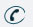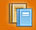781-366-0662

Subject

## AP Calculus BC

AP Calculus BC begins with a brief review of functions, limits, continuity, a brief extension of derivatives and their applications, and continues with integration. Topics include the calculus of polynomial, rational, exponential, trigonometric, polar, and parametric functions; the calculus of vectors; infinite series; and an introduction to differential equations. Students successfully completing this course will be prepared to take the Advanced Placement Calculus BC.

## AP Calculus AB

AP Calculus AB begins the study of calculus and covers functions, limits, continuity, derivatives and integration and their applications. Topics include the calculus of polynomial, rational, exponential and trigonometric functions; and an introduction to differential equations and slope fields. Students who successfully complete this course will be prepared to take the advanced Placement Calculus AB exam offered in May. Graphing calculators are used extensively in this course.Subject Classes